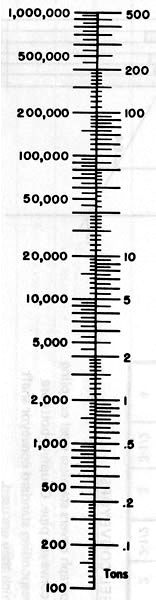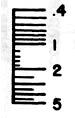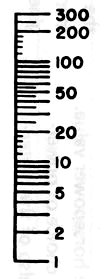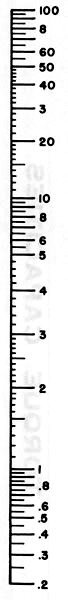## Graphic Method of Calculation

The total horsepower (TSHP) required at the drive shaft to drive the loaded conveyor system may be calculated graphically by use of the nomographs at the end of this section. The friction horsepower (FHP), determined with the first nomograph, added to the Material Horsepower (MHP), determined with the second nomograph, equals the Total Shaft Horsepower (TSHP).

Friction Horsepower – A straight edge placed at the first two known values, conveyor size (related to hanger bearing class as listed in hanger bearing factor table) and length, will intersect a reference point on the centerline. A straight edge placed from this reference point to the third known value, conveyor speed, will intersect the unknown value, Friction Horsepower, on the last line.

Material Horsepower – A straight edge placed at the first two known values, conveyor capacity and Material Horsepower Factor, will intersect a reference point on the centerline. A straight edge from the reference point to the third known value, conveyor length, will intersect the unknown value, Material Horsepower, on the last line.

## Calculation by Equation

TSHP may also be calculated by equation using the following formulas:

## Calculation by Equation

TSHP may also be calculated by equation using the following formulas:

## Material H.P. Calculation:

### OR

Note: If calculated Material Horsepower is less than 5 it should be corrected for potential overload. The corrected horsepower value corresponding to the calculated Material Horsepower will be found on the lower scale of the Material. Horsepower Overload Correction Chart.

## Total Shaft H.P. Calculation

TSHP = FHP + MHP*

*Corrected if below 5 HP.

Note: The actual motor horsepower required to drive the loaded conveyor system is dependent on the method used to reduce the speed the motor to the required speed of the conveyor. Drive losses must be taken into consideration when selecting the motor and drive equipment.

## Equation Symbols

TSHP Total Shaft H.P. Friction H.P. (H.P. required to drive the conveyor empty) Material H.P. (H.P. required to move the material) Conveyor Length Conveyor Speed Conveyor Diameter Factor Hanger Bearing Factor Conveyor Capacity Weight per cu. ft. Capacity, lbs. per hr. Material H.P Factor (From the Materials Table)

Diameter Factor

4

12

6

18

9

31

10

37

12

55

14

78

16

106

18

135

20

165

24

235

## Hanger Bearing Factors

Bearing Type Bearing Factor Bearing Class
Ball

1.0

I

Babbit

1.7

II

Bronze
*Graphite Bronze
Plastic, laminated fabric-base
Nylon
* Bronze, oil-impregnated
Wood
*Plastic, graphite- impregnated

2.0

III

*Nylon
*Teflon
*Hard Iron

4.4

IV

*Hard-Surfaced

*Non-Lubricated

## Conveyors With Modified Flights

The procedure for calculation of horsepower for conveyors with special or modified flights is identical to that used for standard conveyors except that the Material Horsepower must be multiplied by one or more of the following applicable factors.

## Modified Flight Factors

15 30 45 95
Cut Flight

1.10

1.15

1.2

*

Cut & Folded Flight

*

1.50

1.7

*

Ribbon Flight

1.05

1.14

1.20

*

Factor 1 2 3 4
1.29 1.58 1.87 2.16

* Std. paddles at 45° reverse pitch

Total Shaft Horsepower (TSHP) is calculated by adding Material Horsepower, multiplied by the appropriate modified flight factor or factors, to Friction Horsepower.

Note: Conveyors which have deviation in pitch only do not require special consideration, and their horsepower calculations are as described for standard conveyors.

## Example

A 10-inch conveyor 35 feet long with a capacity of 10 tons per hour at 45 RPM has been selected.

From the Materials Table, a Horsepower Factor of 0.8 is found for the material to be conveyed. The table also indicates Series 4 hanger bearings and shafts. Hard iron bearings and hardened coupling shafts have been selected to suit this requirement.

Friction Horsepower, the horsepower required to drive the conveyor empty, is calculated as follows:

Diameter Factor = 37

Hanger Bearing Factor = 4.4

Length = 35

RPM = 45

Since the calculated Material Horsepower is less than 5, it is necessary to find the corrected horsepower value corresponding to 0.56 horsepower on the Overload Correction Chart below. This value is found to be 1.320 horsepower.

Total Shaft Horsepower (TSHP) is the sum of Friction horsepower and the corrected Material Horsepower. Thus TSHP is calculated as follows:

TSHP = 0.256 + 1.320 = 1.576 H.P.

Assuming a drive efficiency of 85% resulting in a total drive horsepower of 1.853, a standard 2 horsepower motor would be selected for the drive input.

The horsepower required for the above conveyor may also be determined graphically by the use of the two horsepower nomographs. The first nomograph determines Friction Horsepower. The second determines Material Horsepower. Total Shaft Horsepower is determined by adding the two values.

Friction Horsepower Material Horsepower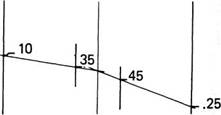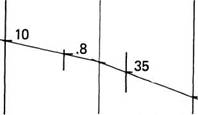Material Horsepower, the horsepower required to move the material, is calculated by the following equation:

Capacity (in lbs. per hr.) = 20,000
Horsepower Factor Length RPM = 4.4
Length = 35
RPM = 45

## Corrected Material H.P.## Friction Horsepower Nomograph

 Bearing Class / Conveyor Size Length Speed Friction H.P.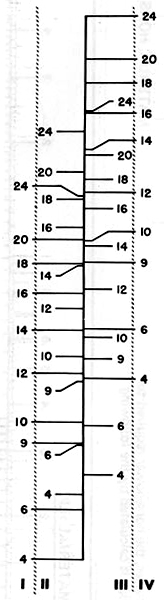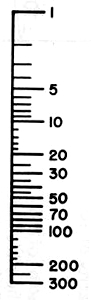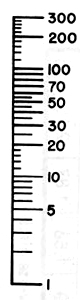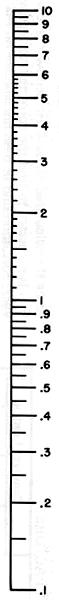## Material Horsepower Nomograph

 Capacity Per Hour Material H.P. Factor Length Material H.P.